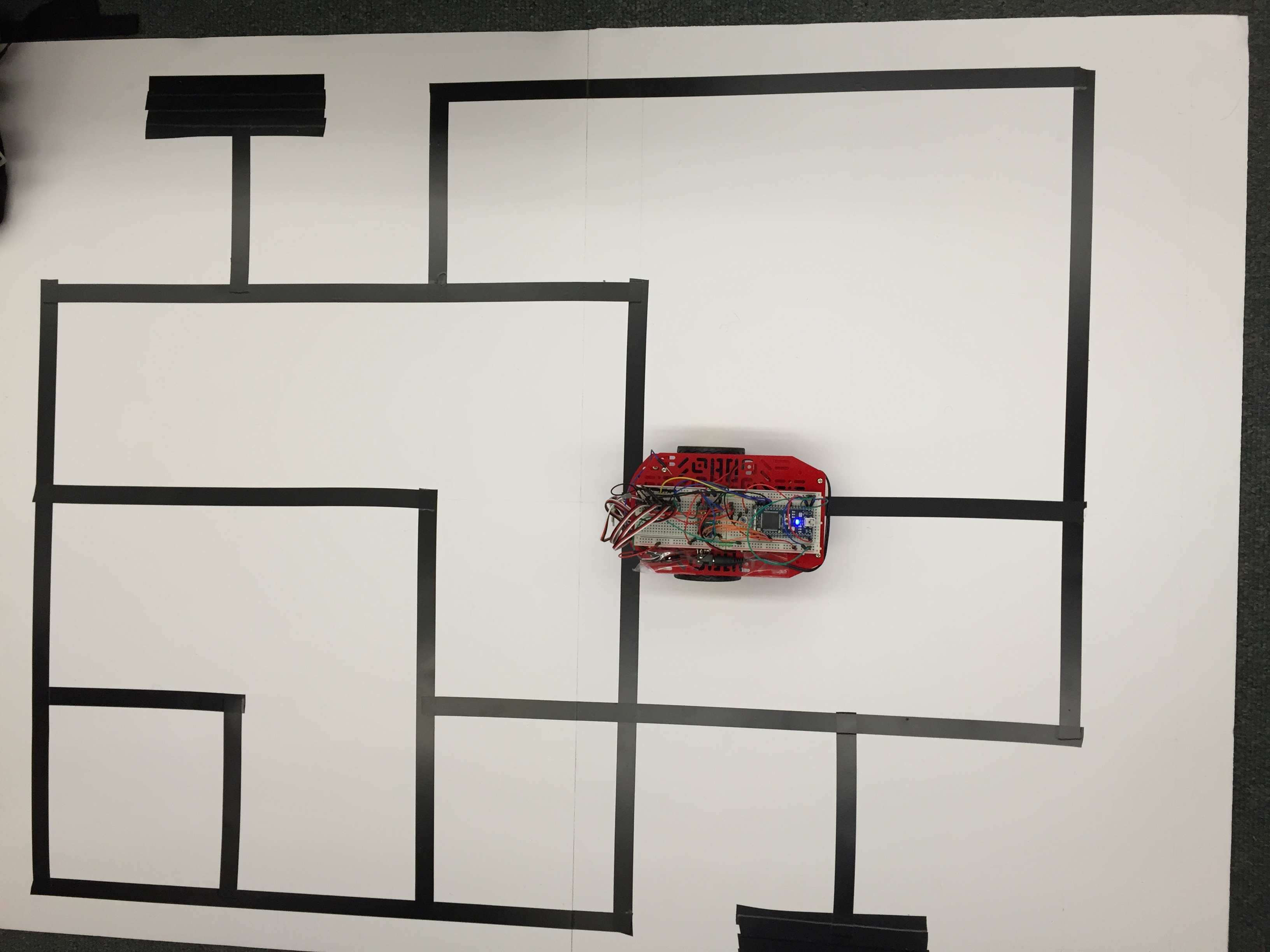# Overview

This project is a maze solving robot. The mbed is connected to the H bridge to control the motors of the Magic Robot. There are 5 lights sensors at the bottom of the robot to sense recognize the black electrical tape. The robot is expected to follow the black tape and complete the maze.

# Hardware Connections

MbedH Bridge
VinVmot
GndGnd
VoutVcc
P21PWMB
P22BIN2
P23BIN1
P24AIN1
P25AIN2
P26PWMA
VoutSTBY
H BridgeRobot DC Motors
A01Left-Red
A02Left-Black
B02Right-Black
B01Right-Red
LightSensorMbed
Pin 1P15,P16,P17,P18,P19
Pin 26V power Supply
Pin 3GND
MbedPushButtons
GndPin 2
P8Pin 1

# Robot# Program

```#include "mbed.h"
#include "motordriver.h"
#include <string>

//See http://mbed.org/cookbook/Motor
//Connections to dual H-brdige driver for the two drive motors
Motor  left(p21, p22, p23, 1); // pwm, fwd, rev, has brake feature
Motor right(p26, p25, p24, 1);

DigitalIn s1(p15);
DigitalIn s2(p16);
DigitalIn s3(p17);
DigitalIn s4(p18);
DigitalIn s5(p19);
//Serial pc(USBTX,USBRX) ;

int p = 0.5 ;
string s1b;
string s2b;
string s3b;
string s4b;
string s5b;
string combined;
string combined2;
int straight;
string path = "";
int U = 0 ;
int finish = 0 ;
int fline = 0 ;
// less than 0.5 == white
// more than 0.5 == black

void convbin()
{
if (s1<=p) s1b = '0';
else s1b = '1';
if (s2<=p) s2b = '0' ;
else s2b = '1';
if (s3<=p) s3b = '0' ;
else s3b = '1';
if (s4<=p) s4b = '0' ;
else s4b = '1';
if (s5<=p) s5b = '0' ;
else s5b = '1';

combined = s1b + s2b + s3b + s4b + s5b ;
//    pc.printf("%s", combined) ;
}

#define intersections

#ifdef intersections
void inch()   //moves an inch
{
left.speed(0.4) ;
right.speed(0.4) ;
wait (0.4) ; //ADJUST THIS so it moved an tape length
left.speed(0) ;
right.speed(0) ;
convbin() ;
if (combined == "00000" ) straight = 0 ;
else if (combined == "11111") fline = 1;
else straight = 1 ;
}

#endif

int main()
{
while(finish == 0) {
convbin() ;
left.speed(0.4);
right.speed(0.4);
wait (0.01) ;
left.speed(0);
right.speed(0);
} else if (combined == "01100" || combined == "01000") { //robot on the left
left.speed(0.4) ;
right.speed(0.3) ;
wait (0.01) ;
left.speed(0);
right.speed(0);
} else if (combined == "00110") { //robot on the right
left.speed(0.3) ;
right.speed(0.4) ;
wait (0.01) ;
left.speed(0);
right.speed(0);
} else if (combined == "11000") { //robot on the left left
left.speed(0.4) ;
right.speed(0.2) ;
wait (0.01) ;
left.speed(0);
right.speed(0);
} else if (combined == "10000") { //robot on the left left left
left.speed(0.2) ;
right.speed(0.4) ;
wait (0.01) ;
left.speed(0);
right.speed(0);
} else if (combined == "00011") { //robot on the right right
left.speed(0.4) ;
right.speed(0.2) ;
wait (0.01) ;
left.speed(0);
right.speed(0);
} else if (combined == "00001") { //robot on the right right right
left.speed(0.4) ;
right.speed(0.2) ;
wait (0.01) ;
left.speed(0);
right.speed(0);
}
#ifdef intersections
else {
combined2 = combined ;
if (combined == "00111" || combined == "01111") {
wait(1);
inch(); //subroutine to go one more inch forward
wait(1);
if (straight == 0) {  //right turn only
left.speed (-0.4) ;
right.speed (0.4) ;
wait (0.7) ; // ADJUST THIS
left.speed(0) ;
right.speed(0);
wait(1);
} else { //both right and straight
left.speed (0.4) ;
right.speed (0.4) ;
path = path + 'S' ;
left.speed(0) ;
right.speed(0);
wait(1);
}
} else if (combined == "11100" || combined == "11110") {
wait(1);
inch() ;
wait(1);
left.speed (0.4) ;
right.speed (-0.4) ;
wait (0.7) ; // ADJUST THIS
left.speed(0) ;
right.speed(0);
wait(1);
if (straight == 1 ) { //left and straight available
path = path + 'L' ;
}

} else if (combined == "11111") {
wait(1);
inch() ;
wait(1);
if (fline == 1) finish = 1 ; //FINISH!!!
else{ //left right straight intersection
left.speed (0.4) ;
right.speed (-0.4) ;
path = path + 'L' ;
left.speed(0) ;
right.speed(0);
wait(1);
}
} else if (combined == "00000") {
left.speed (0.4) ;
right.speed (-0.4) ;
path = path + 'U' ;
left.speed(0);
right.speed(0);
wait(1);
}
}

#endif
}

}
```

# Team Members

 Carlos Del Rio Sanjay Ravi Greg Roberts Ramon Sua